# Parallelogram - Definition with Examples

The Complete Prek-5 Learning Program Built for Your Child
Home > Parallelogram

What is Parallelogram?

A parallelogram is a special type of quadrilateral that has equal and parallel opposite sides.

The given figure shows a parallelogram ABCD which as AB parallel to CD and AD parallel to BC.

Also, AD = BC and AB = CD.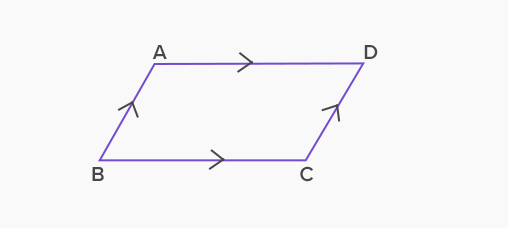We also see a lot of parallelogram like shapes and objects around us.

Properties of parallelogram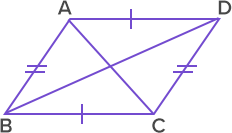The opposite sides of a parallelogram are parallel to each other.  Here, AB ∥ CD and AD ∥ BCThe opposite sides of a parallelogram are equal in length.  Here, AB = CD and AD = BC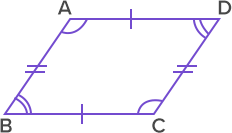The opposite angles of a parallelogram are equal in measure.  Here, Angle A = Angle C and  Angle B = Angle D.Adjacent angles of a parallelogram add up to 180°  Here, Angle A + Angle B = 180°  Angle B + Angle C = 180°  Angle C + Angle D = 180°  Angle D + Angle A = 180°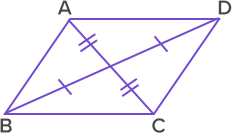Diagonals of a parallelogram bisect each other.  Here AC and BD bisect each other.

Types of a parallelogram

There are three special types of a parallelogram.

1. Rhombus: A parallelogram in which all sides are equal.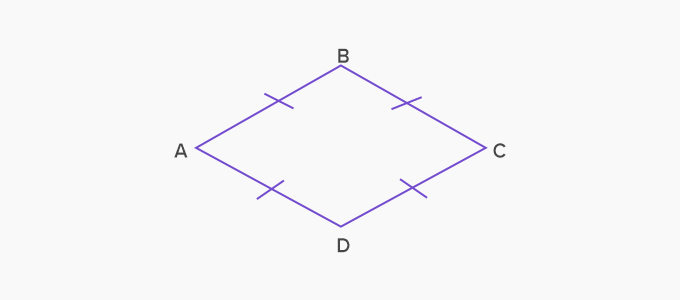Here AB = BC = CD = DA.  ABCD is a rhombus.

2. Rectangle: A parallelogram in which all angles are right angles and the diagonals are equal.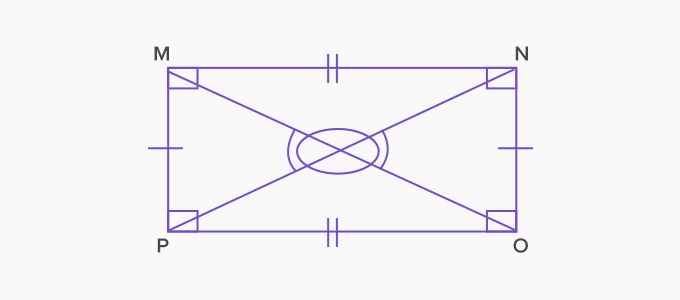Here all angles are right angles. Diagonals PN and OM are equal.

3. Square: A parallelogram with all equal sides and all angles equal to 90 degrees. The diagonals of a square are also equal.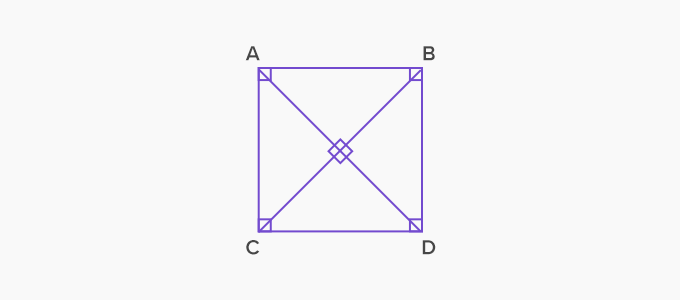Here all sides are equal and all the angles are right angles.

Diagonals AC and BD are equal.

 Fun Facts The term ‘parallelogram’ is derived from Middle French ‘parallélogramme’, Late Latin ‘parallelogrammum’ and Greek ‘parallelogrammon’ which means “bounded by parallel lines”.